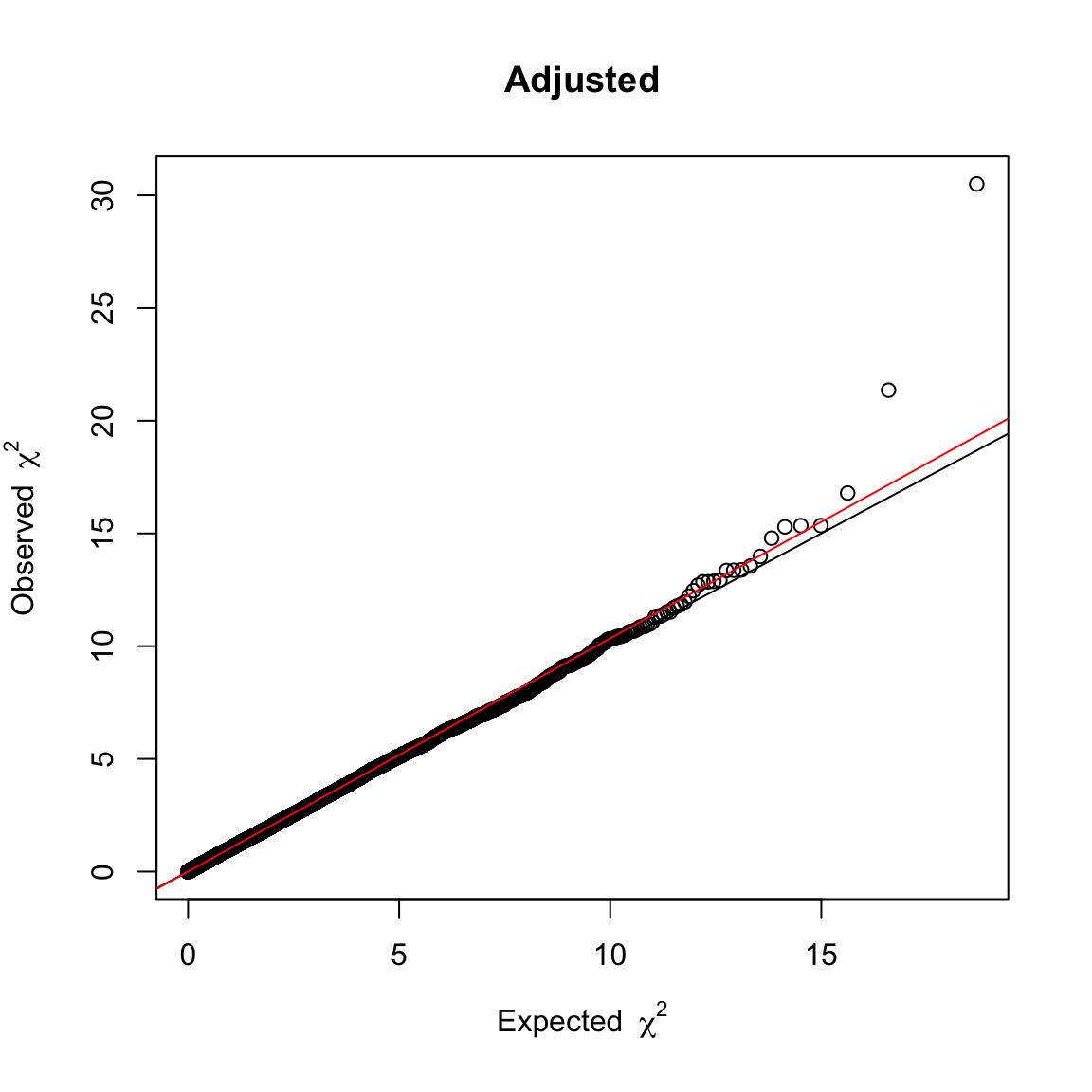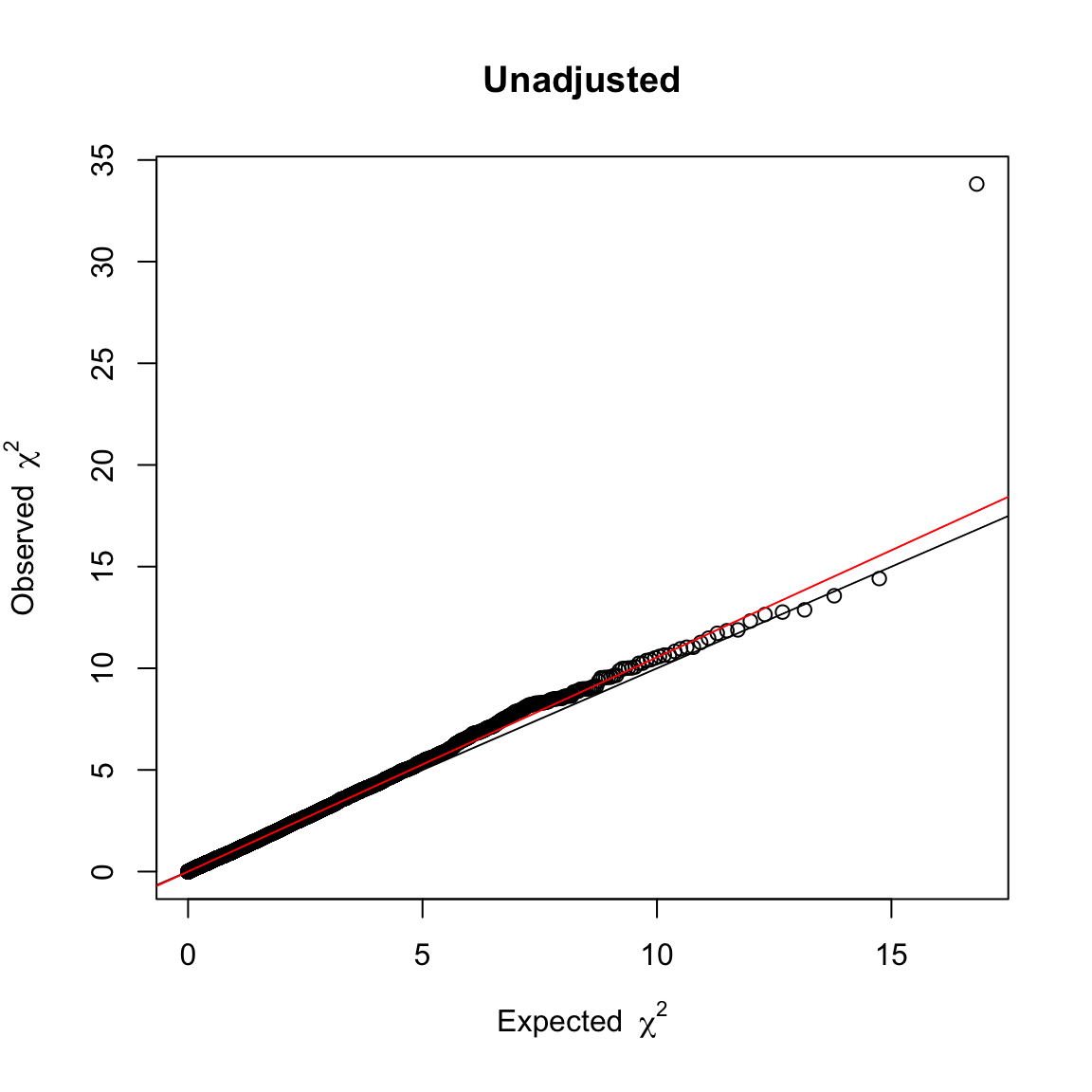## Q-Q plots and the $$\lambda$$-statistic

(This lab was adapted for statsTeachR by Sara Nunez, Nicholas Reich and Andrea Foulkes from our GWAS tutorial.)

In the next several labs, we go over methods for data visualization and post-analytic quality control checks. In the current assignment, we will walk through how to generate Q-Q plots and calculate lambda statistics to determine if our results are influenced by confounding.

### Getting started

To start off, as usual, we load the data from previous labs and necessary libraries:

library(doParallel)
library(snpStats)
library(GenABEL)
load("m4_lab1_save.RData")

## Q-Q plots

Q-Q plots are used to visualize the relationship between the expected and observed distributions of SNP level test statistics. Largely, it can be used to determine if the results were influenced by unaccounted for confounding effects. Here, to demonstrate how this might work, we compare the test statistics from the model we fit previoulsy, adjusted for principal components and other clincally relevant covariates, with a new model generated with only participant ID and SNP as predictors. We then plot the test statistics from the two models utilizing the function estlambda() from the GenABEL package.

First, we need to fit a new model that doesn’t account for covariates for comparative purposes. To keep it simple, we will only focus on chromosome 16 in this lab. If you recall, the imputation lab only considered SNPs on chromosome 16, and stored their genotypes in the ‘target’ object. In the following code, we use this fact to subset the output from the original GWAA for SNPs on chromosome 16, and then subset the genotype data for the 1308 individuals that passed sample quality control checks. We also go ahead and subset the phenotype data so that it only includes columns for ‘id’ and ‘phenotype.’

gwas1 <- GWASout[GWASout$SNP%in%colnames(target),] genosub <- target[as.character(phenodata$id),]

phenosub <- phenodata[,c("id","phenotype")]

#### Exercise

How many SNPs are on chromosome 16? Are the number of SNPs in gwas1 and genosub equal? Why or why not?

#### Exercise

What information from gwas1 will we be using and why?

Next, we need to rerun the analysis with ‘id’ as the only confounding variable included in the model. To do this, we need to go through the same motions as before, in which we split the data up into smaller subets, and initialize a .txt file for the results to be written to. We then run the new analysis and store the results in a file named ‘GWAA_unadj.txt.’

nSNPs <- ncol(genosub)
nSplits <- 10
genosplit <- ceiling(nSNPs/nSplits) # number of SNPs in each subset
snp.start <- seq(1, nSNPs, genosplit) # index of first SNP in group
snp.stop <- pmin(snp.start+genosplit-1, nSNPs) # index of last SNP in group

columns<-c("SNP", "Estimate", "Std.Error", "t-value", "p-value")

gwas2 <- GWAA(genodata=genosub, phenodata=phenosub, filename="GWAA_unadj.txt")
## socket cluster with 2 nodes on host 'localhost'
## GWAS SNPs 1-1217 (10% finished)
## GWAS SNPs 1218-2434 (20% finished)
## GWAS SNPs 2435-3651 (30% finished)
## GWAS SNPs 3652-4868 (40% finished)
## GWAS SNPs 4869-6085 (50% finished)
## GWAS SNPs 6086-7302 (60% finished)
## GWAS SNPs 7303-8519 (70% finished)
## GWAS SNPs 8520-9736 (80% finished)
## GWAS SNPs 9737-10953 (90% finished)
## GWAS SNPs 10954-12164 (100% finished)
##  "Done."

Now that our results are stored, we can read them in and plot the results. This will require plotting the squared test statistics (which follow a $$\chi^2$$ distribution) for both the adjusted and unadjusted models. Note that because there are so many observations, the plots are displayed rather large so that the details of the plots are visible.

# read in model results
rep("numeric", 4)))

# QQ plot for adjusted model
lambdaAdj <- estlambda(GWASout$t.value^2, plot = TRUE, method = "median", main = "Adjusted")# QQ plot for unadjuested model lambdaUnadj <- estlambda(GWASoutUnadj$t.value^2, plot = TRUE, method = "median",
main = "Unadjusted")#### Exercise

What do you observe? What do these plots tell you about the variables included in the adjusted model that were left out of the unadjusted model?

### $$\lambda$$-statistics

Another way to determine whether or not there is confounding effecting your results is to look at the $$\lambda$$ statistics for each model. This can be obtained from the ‘estimate’ parameter of the above stored objects from estlambda(). Deviations from 1 imply confounding, although deviations tend to be small in this context.

c("Unadjusted model lambda:", lambdaUnadj$estimate) ##  "Unadjusted model lambda:" "1.05405027919361" c("Adjusted model lambda:", lambdaAdj$estimate)
##  "Adjusted model lambda:" "1.03449915443322"

While both statistics are very close to 1, the unadjusted model has a slight larger deviation.

#### Exercise

Recall that the majority of our covariates were principal components to adjust for population substructure in the original model. How might this explain $$\lambda$$ statistics with such small deviations from 1?

### Saving work for following labs…

Although we won’t need the data generated in this lab for future labs, we will resave the previous data, as it will be needed again:

save(imputed, target, rules, phenodata, support, genes, impCETP, impCETPgeno,
imputeOut, GWAA, map2gene, GWASout, GWAScomb, CETP, file = "m4_lab2_save.RData")